# What is the Time? (3)

In this worksheet, students practise telling the time from an analogue clock face.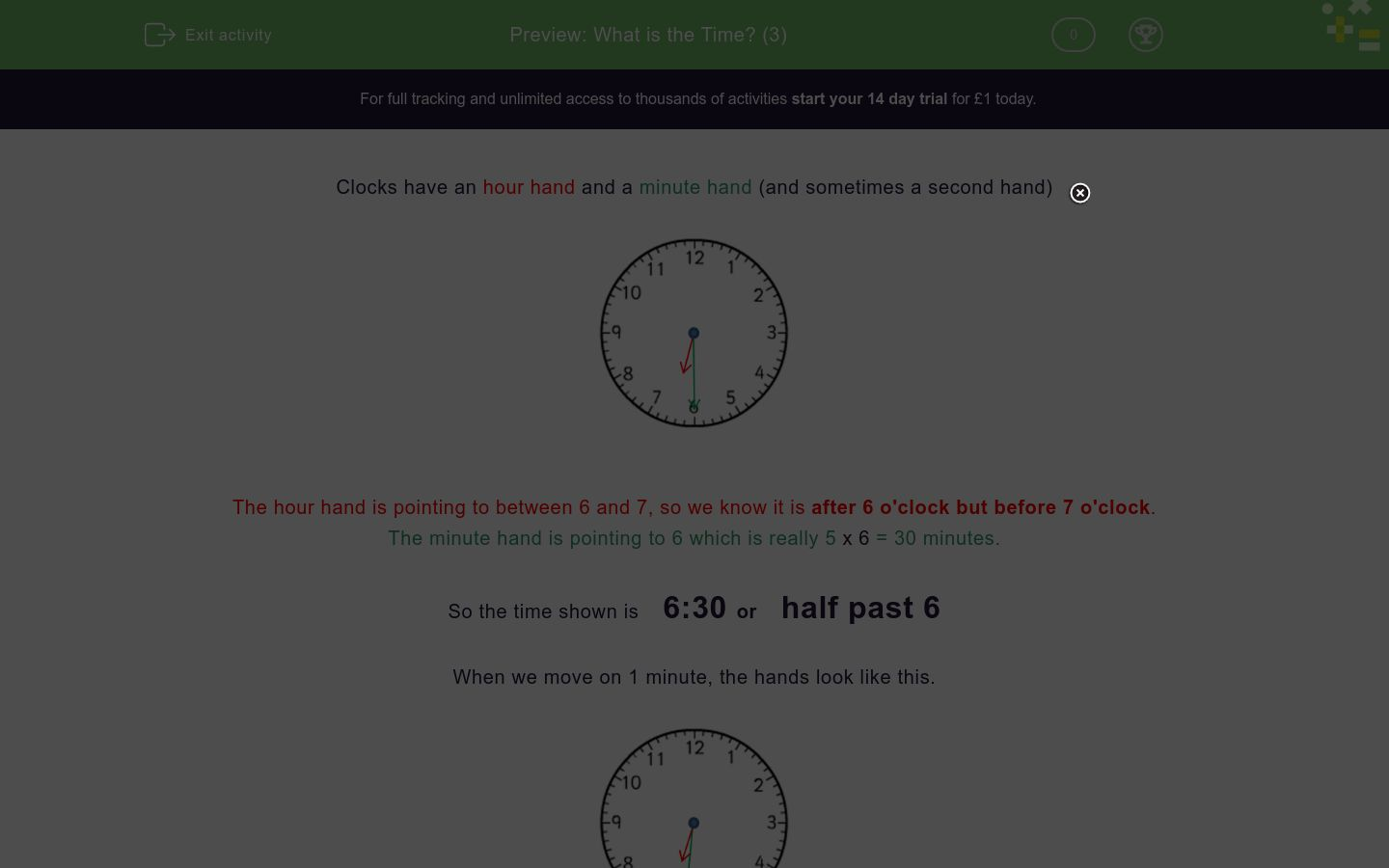Key stage:  KS 2

Curriculum topic:   Measurement

Curriculum subtopic:   Tell the Time (analogue, 12-hour clocks, 24-hour clocks)

Difficulty level:### QUESTION 1 of 10

Clocks have an hour hand and a minute hand (and sometimes a second hand)The hour hand is pointing to between 6 and 7, so we know it is after 6 o'clock but before 7 o'clock.

The minute hand is pointing to 6 which is really 5 x 6 = 30 minutes.

So the time shown is    6:30 or    half past 6

When we move on 1 minute, the hands look like this.The hour hand has moved a tiny tiny tiny amount.

The minute hand has moved forward one minute.

So the time shown is    6:31

Want to understand this further and learn how this links to other topics in maths?
Why not watch this video?

What is the exact time, shown on this clock face?6:31

6:29

6:30

What is the exact time, shown on this clock face?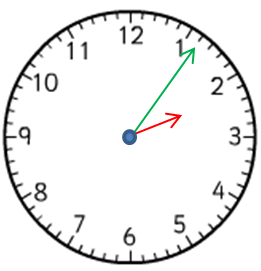2:11

2:05

2:06

What is the exact time, shown on this clock face?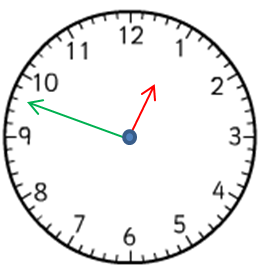12:48

12:09½

12:45

What is the exact time, shown on this clock face?12:50

10:03

10:12½

What is the exact time, shown on this clock face?3:09

3:47

9:17

What is the exact time, shown on this clock face?5:56

11:28

11:27

What is the exact time, shown on this clock face?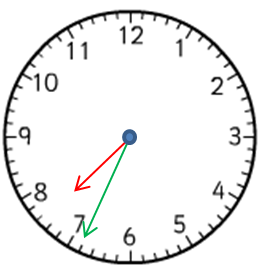7:08

7:34

7:07

What is the exact time, shown on this clock face?What is the exact time, shown on this clock face?What is the exact time, shown on this clock face?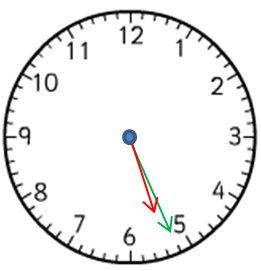• Question 1

What is the exact time, shown on this clock face?6:29
• Question 2

What is the exact time, shown on this clock face?2:06
• Question 3

What is the exact time, shown on this clock face?12:48
• Question 4

What is the exact time, shown on this clock face?10:03
• Question 5

What is the exact time, shown on this clock face?9:17
• Question 6

What is the exact time, shown on this clock face?11:27
• Question 7

What is the exact time, shown on this clock face?7:34
• Question 8

What is the exact time, shown on this clock face?8:56
• Question 9

What is the exact time, shown on this clock face?4:13
• Question 10

What is the exact time, shown on this clock face?5:26
---- OR ----

Sign up for a £1 trial so you can track and measure your child's progress on this activity.

### What is EdPlace?

We're your National Curriculum aligned online education content provider helping each child succeed in English, maths and science from year 1 to GCSE. With an EdPlace account you’ll be able to track and measure progress, helping each child achieve their best. We build confidence and attainment by personalising each child’s learning at a level that suits them.

Get started